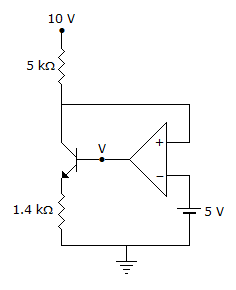# Electronics and Communication Engineering - Exam Questions Papers

41.

For a message signal m(t) = cos (2pfmt) and the carrier frequency fc, which of the following represents a single side band (SSB) signal?

 A. cos (2pfmt) cos (2pfct) B. cos (2pfct) C. cos [2p(fc + fm) t] D. [1 + cos (2pfmt) cos (2pfct)]

Explanation:

No answer description available for this question. Let us discuss.

42.

In the circuit shown below, the op-amp is ideal, the transistor has VBE = 0.6 V and β = 150. Decide whether the feedback in the circuit is positive or negative and determine the voltage V at the output of the op-ampA. Positive feedback, V = 10 V B. Positive feedback, V = 0 V C. Negative feedback, V = 5 V D. Negative feedback, V = 2 V

Explanation:

No answer description available for this question. Let us discuss.

43.

Which of the following addressing modes is used in the instruction PUSH B?

 A. Direct B. Register C. Register Indirect D. Immediate

Explanation:

No answer description available for this question. Let us discuss.

44.

Consider a silicon p-n junction at room temperature having the following parameters:
Doping on the n-side = 1 x 1017 cm-3
Depletion width on the n-side = 0.1 μm
Depletion width on the p-side = 1.0 μm
Intrinsic carrier concentration = 1.4 x 1014F. cm-1.
Thermal voltage = 26mV
Permittivity of free space = 8.85 x 10-14F. cm-1.
Dielectric constant of silicon = 12. The peak electric field in the device is

 A. 0.15 MV.com-1, directed from p-region to n-region B. 0.15 MV.com-1, directed from n-region to p-region C. 1.80 MV.com-1, directed from p-region to n-region D. 1.80 MV.com-1, directed from n-region to p-region

Explanation:

No answer description available for this question. Let us discuss.

45.

A single turn loop is situated in air, with a uniform magnetic field normal to its plane. The area of the loop is 5 m2 and the rate of change of flux density is 2 Wb/m2/s. What is the emf appearing at the terminals of the loop?

 A. -5 V B. -2 V C. -0.4 V D. 0 V